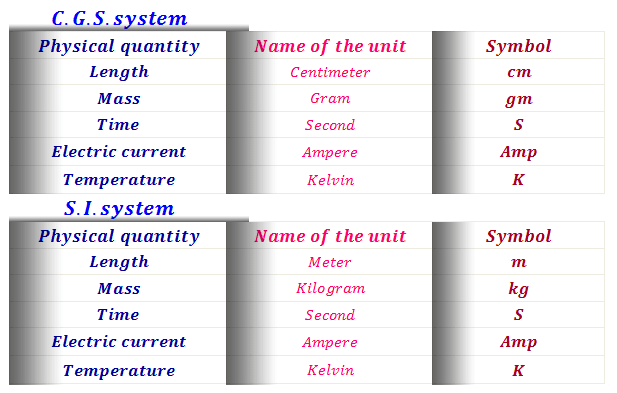Unit dimension for students

Physical quantities

In physical chemistry, we commonly deal with quantities such as pressure, volume, mass, temperature, current, etc. These quantities are known as physical quantities.
A physical quantity has two components, namely, numerical value and it's unit and is written as,
The quantity 5 Newton means the physical quantity Newton and its numerical value 5Unit dimension
The dimension of length = L, mass = M, and time = T.

Definition of base physical quantities

Meter

The meter is the length of the path traveled by light in vacuum during a time interval of 1/299 792 458 of a second.

Unit and dimension of the area

Area = length × length
Thus the unit of area = unit of length × unit of length

Unit of area in C.G.S. system = cm × cm
= cm²

Unit of the area in S.I. the system is = m × m
= m²

Dimension of area = L × L
= [L]²

Unit and dimension of Volume

Volume (V) = length × length × length.
Thus the unit of volume = length × length × length

Unit of area in C.G.S. system = cm × cm × cm
= cm³

Unit of the area in S.I. the system is = m × m × m
= m³

The dimension of area = L × L × L
= [L³]

Unit and dimension of density

From the definition of density (d) = mass/volume

Thus the unit of density = unit of mass/unit of volume

Unit of area in C.G.S. system = gm/cm³
= gm cm⁻³

Unit of the area in S.I. system is = Kg/m³
= Kg m⁻³
Dimension of area = [M]/[L³]
= [M L⁻³]

Unit and dimension of force

From the Newton second law of motion,
force = mass × acceleration
or, force = mass × velocity/time
or, force = mass × (length/time)/time

Here velocity = length/time

∴ Force = mass × (length/time²)

Thus the unit of force in C.G.S. system,
= unit of mass × (unit of length/unit of time²)
= gm × cm/sec² = gm cm/sec² or, gm cm sec⁻²
or, Dyne

Again in the same way unit of force in S.I system,
= Kg m/sec²
or, Kg m sec⁻²
or, Newton
From the above discussion dimension of force, = dimension of mass × dimension of length/dimension of time².
Thus the dimension of force
= [M L T⁻²]
Problem
How to convert 1 newton into a dyne?
1 Newton = Kg m/sec²
= (1 Kg× 1 m )/(1 sec)²

We know that 1 Kg = 10³ gm and 1 m = 10² cm

Thus, 1 Newton = (10³gm × 10² cm)/(1 sec)²
= 10⁵ gm cm/sec2

= 10⁵ Dyne

Unit and dimension of work

From the definition of work (W)
= force(F) × displacement(d).
Thus, the unit work = unit of force × unit of displacement(d)

∴ Unit of work in C.G.S. system, = (gm cm/sec²) × cm
or, gm cm²/sec²
or, gm cm²sec⁻²
or, erg

And the unit of work in S.I. system = (Kg m/sec²) × m
or, Kg m²/sec²
or, Kg m²sec⁻²
or, Joule
From the above discussion dimension of work, = (dimension of mass × dimension of length)²)/(dimension of time²)
Thus the dimension of work
= [ML²T⁻²]
Problem
How to convert 1 joule into erg?
1 Joule = 1 Kg m²/sec²
= (1 Kg × 1 m² )/1 sec²

We know that 1 Kg = 10³ gm and 1 m = 10² cm
Thus, 1 Joule = {10³ gm × (10²)² cm}/1 sec²
= 10⁷ gram cm²/sec²
= 10⁷ erg

Unit and dimension of Force

The ability to doing work is termed as energy. Thus, the unit and dimension of energy and work are the same.
The unit of energy in the C.G.S system is erg and S.I. system is Joule

Unit and dimension of pressure

From the definition of pressure (P) = force/area

Thus the unit of pressure = unit of force/Unit of area.

Unit of pressure in C.G.S. System = erg/cm²
= erg cm⁻²

Unit of pressure in S.I. system is = joule/m²
= joule m⁻²

Dimension of pressure
= [M L⁻²]

Unit dimension of physical quantities, physical quantities, units and dimension of the area, volume, density, force, work, pressure